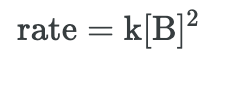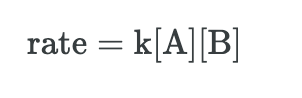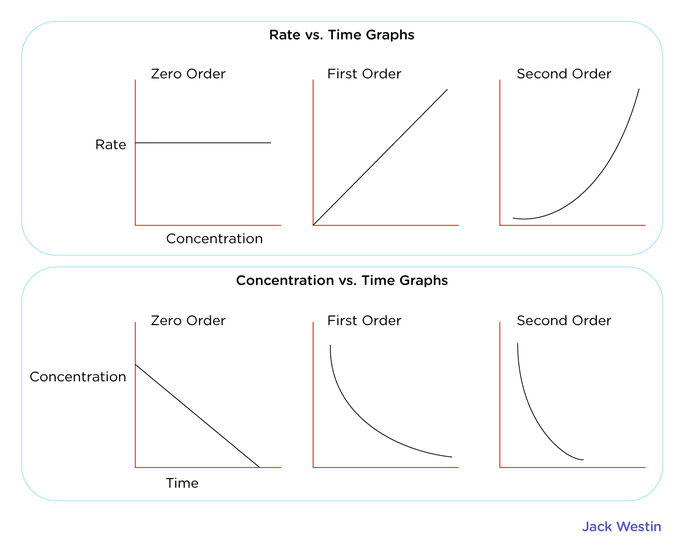Complimentary 1-hour tutoring consultation

MCAT Content / Rate Processes In Chemical Reactions Kinetics And Equilibrium / Dependence Of Reaction Rate On Concentration Of Reactants

### Dependence of Reaction Rate on Concentration of Reactants

Topic: Rate Processes In Chemical Reactions Kinetics And Equilibrium

When the concentrations of the reactants are raised, the reaction proceeds more quickly.

Raising the concentrations of reactants makes the reaction happen at a faster rate. For a chemical reaction to occur, there must be a certain number of molecules with energies equal to or greater than the activation energy. With an increase in concentration, the number of molecules with the minimum required energy will increase, and therefore the rate of the reaction will increase.

The rate law for a chemical reaction is an equation that relates the reaction rate with the concentrations or partial pressures of the reactants. For the general reaction:

aA+bB→C

With no intermediate steps in its reaction mechanism, meaning that it is an elementary reaction, the rate law is given by:

r=k[A]x[B]y

In this equation, [A] and [B] express the concentrations of A and B, respectively, in units of moles per liter. The exponents x and y vary for each reaction, and they must be determined experimentally; they are not related to the stoichiometric coefficients of the chemical equation. Lastly, k is known as the rate constant of the reaction. The value of this coefficient k will vary with conditions that affect the reaction rate, such as temperature, pressure, surface area, etc. A smaller rate constant indicates a slower reaction, while a larger rate constant indicates a faster reaction.

The reaction order is the relationship between the concentrations of species and the rate of a reaction. To work out x and y in the rate equation we need to consider experimental data using the concentrations of A and B and examining the effect they have on the rate of reaction. x and y are known as orders and refers to the power dependence of the rate on the concentration of each reactant.

r=k[A]x[B]y

A first-order reaction depends on the concentration of only one reactant. As such, a first-order reaction is sometimes referred to as a unimolecular reaction. While other reactants can be present, each will be zero-order, since the concentrations of these reactants do not affect the rate. Thus, the rate law for an elementary reaction that is first order with respect to a reactant A is given by:

A second-order reaction is when the overall order is two. For a reaction with the general form: aA+bB→C the reaction can be second order in two possible ways. It can be second-order in either A or B, or first-order in both A and B. If the reaction were second-order in either reactant, it would lead to the following rate laws:The second scenario, in which the reaction is first-order in both A and B, would yield the following rate law:For a zero-order reaction, increasing the concentration of the reacting species will not speed up the rate of the reaction. Zero-order reactions are typically found when a material that is required for the reaction to proceed, such as a surface or a catalyst, is saturated by the reactants.Practice Questions

MCAT Official Prep (AAMC)

Chemistry Online Flashcards Question 1

Chemistry Question Pack Passage 10 Question 56

Practice Exam 1 C/P Section Passage 9 Question 51

Practice Exam 2 C/P Section Passage 7 Question 35

Practice Exam 3 C/P Section Passage 6 Question 31

Key Points

• When the concentrations of the reactants are raised, the reaction proceeds more quickly. This is due to an increase in the number of molecules that have the minimum required energy.

• For a generic reaction aA+bB→C with no intermediate steps in its reaction mechanism (that is, an elementary reaction), the rate is given by: r=k[A]x[B]y

• The rate equation of a reaction with a multi-step mechanism cannot, in general, be deduced from the stoichiometric coefficients of the overall reaction; it must be determined experimentally.

• The order of a reaction refers to the power dependence of the rate on the concentration of each reactant and a reaction can be 0, 1 or 2 order with respect to the reactants and its effect on the rate

Key Terms

Activation energy: The minimum energy required for a reaction to occur.

Rate law: An equation relating the rate of a chemical reaction to the concentrations or partial pressures of the reactants.

Reaction mechanism: The step-by-step sequence of elementary transformations by which overall chemical change occurs.

Rate constant: A coefficient of proportionality relating the rate of a chemical reaction at a given temperature to the concentration of reactant (in a unimolecular reaction) or to the product of the concentrations of reactants.

First-order reaction: A reaction that depends on the concentration of only one reactant (a unimolecular reaction). Other reactants can be present, but each will be zero-order.

Second-order reaction: A reaction that depends on the concentration(s) of one second-order reactant or two first-order reactants.

Zero-order reaction: A reaction that has a rate that is independent of the concentration of the reactant(s).

Billing Information